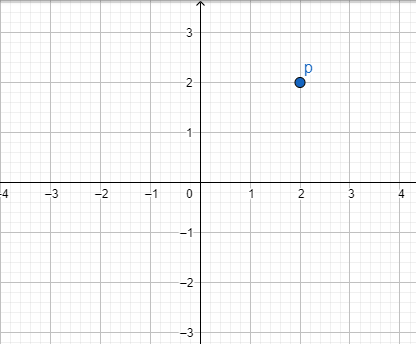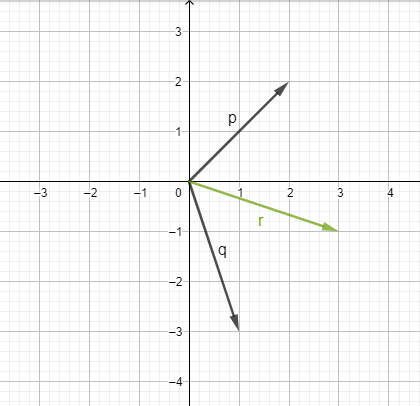Algebra 2 Homework Help | Algebra 2 Tutor | High School Algebra 2

# 24/7 Algebra 2 Homework Help!

Avail written help from TutorEye to gain better conceptual clarity & confidence.

## Basic concepts in Algebra 2:

### Quadratic Equation: -

Any function in the form of ax2+bx+c=0, where a should not be equal to zero is known as quadratic function. It has its degree as 2, which means highest power of a term in that expression should be 2.

### Probability: -

In order to find the possibility or chance or surety of an event we use the concept of probability. It is used to find the chances of occurrence of an event and value of that chance is always in between 0 and 1.

Notation of Probability is PA= number of favourable outome/tatal number of outcomes

### Trigonometry: -

Study of the measure of the three sides and their relationship with angles of triangle. In most of the cases trigonometry is applied in only right-angle triangle. sinθ, cosθ, tanθ, etc are some trigonometric ratio.

## Tips for solving algebra 2 problems - Sample:

Topic: The complex plane

(Easy)

Question: ​​​​The given complex number is,, draw the complex number on the complex plane.

Explanation: A complex number of formmeans, it will beunit on real axis, andunit on the imaginary axis.

The horizontal axis on the complex plane is the real axis, and the vertical axis is the imaginary axis.

Graph:

The complex numberwould be 2 units on the real axis, and 2 units on the imaginary axis.

The location of the complex number would be:

##Topic: Adding and subtracting complex number

(Medium)

Question:  The given complex numberand,, Find the sum of complex numbers such that. And show them on a complex plane as a vector.

Explanation: To add the complex numbers, we need to add the real part and imaginary part separately.

Calculation: FindGraph: The vector connecting the location of point from origin, would represent the corresponding vectors.

##Topic: Multiplying complex number

(Hard)

Question: The given complex numberand,, Find the multiplication of complex numbers such that. And show them on complex plane as a vector.

Explanation: We can multiply the complex number using distribution. Also, we knowCalculation: FindPlugGraph: Plot each vector on the complex plane.

## Fast and Reliable Help For High School Algebra 2

Algebra 2 is the third math course introduced in high school after algebra 1 course. It is also known or referred as intermediate algebra and college algebra. Algebra 2 is considered as a core math course and most of the states in united states including Washington, D.C. has made Algebra 2 a required course for high school graduation.

Normally after algebra 2, Students can move to other advanced math concepts such as pre-calculus and then calculus. Algebra 2 questions are also a part of the SAT exam syllabus.

Algebra 2 has a lot of real-life applications and solving algebra 2 problems can become easy if teachers connect the problems with real world situations. For example - Exponential equations are used widely in day-to-day life for solving savings or loan situations.

Many experts in the field of Math state that high school algebra 2 concepts can help students excel in their career and perform complex tasks on the job with ease even in situations when their job descriptions are not math related.

Algebra 2 is advanced math and may be considered a hard course by high school students due to the complexity of concepts taught in the course. But, Algebra 2 is easy as long as students keep up with their daily Algebra 2 homework and have conceptual clarity.

A lot of times free online Algebra 2 homework help may seem like a good idea but in the long run lack of expert guidance from subject matter experts can lead to bad command of Algebra 2 for high school students.

Take homework help from seasoned Algebra 2 tutors and get transported to a mathematical world of equations. With personalized and detailed solutions to your algebra 2 problems our experts will surely help you conquer the concepts. Our step-by-step explanations and detailed instructions have helped a lot of students around the world.

Our tutors can help you meet a deadline for your Algebra 2 assignment. Get insights on figuring out the Algebra 2 problems and easy hacks to a difficult question. To make studying simple for you, we provide 24/7 written help. Prepare with the best in the field for further advanced work in mathematics.

TutorEye will make you smarter, sharper, and better prepared for the upcoming tests and exams. Get ready for better grades today!

## Follow these simple steps to get Algebra 2 homework answer:

Step 1: Fill in your homework request details on our quick-help form

Step 2: Hire a tutor after duly considering your budget and submission deadline

Step 3: Check the final assignment before releasing money from escrow

Our homework solvers consider each question and provide assignments that are of the highest quality and are 100% original. Meet the top helpers who have devoted their careers helping thousands of students today!

## Top Algebra 2 Homework Help Questions:

### What is algebra 2?

The third Math course in high school is called Algebra 2. It builds on knowledge and skills used while studying Algebra 1. It is taught in 11th grade and focuses on exponentials and logarithmic functions. Students also call it “College Algebra”.

It covers the following topics:

• Complex numbers
• Polynomial graphs
• Logarithms
• Modeling

Therefore, a student must be ready to seek additional study aid from our experts at TutorEye to succeed in this course.

### How hard is algebra 2?

Only if you are not clear with your basics of Algebra 1, you will find Algebra 2 quite hard. As the foundation of this course is built upon the principles you learnt in Algebra 1, you need to have a solid base as such. But you will not find it tough if you revise old topics and continue to expound on them while solving new problems.
Besides, you can always take Algebra 2 help from top professionals at TutorEye to figure out where you should focus to make this course easier for you.

### What do you learn in algebra 2?

Students learn a lot in Algebra 2 specifically they get well-versed in tools modelling the real world. Here your understanding of functions and real numbers is extended.

The learning outcomes include:

• Evaluate different problems
• Factor a polynomial function
• Analyze a function rule
• Solve expressions

Also by doing your assignments you know what topics need more time and effort. Seek algebra 2 homework help at TutorEye to no longer struggle in the subject,

### What does algebra 2 cover?

Algebra 2 covers the following topics:

• Complex numbers
• Exponential models
• Polynomial division and factorization
• Rational exponents

You learn how to do more complex factoring that’s why you must not shy away from taking algebra 2 help from us.

### How to pass algebra 2?

To pass in Algebra 2 you must follow these steps:

• Focus on conceptual understanding
• Relate to real life activities
• Address your difficulties as soon as they arise
• Write down important formulas
• Attend all classroom lectures
• Take additional help from multiple resources
• Practice old questions and exam papers
• Solve your worksheets

If you are still stumped, get in touch with our reputed experts to help you with a challenging curriculum. We will help you in breaking down the syllabus and focus on smaller parts. Build on your success by solving problems daily.

## We Are Different from Others!